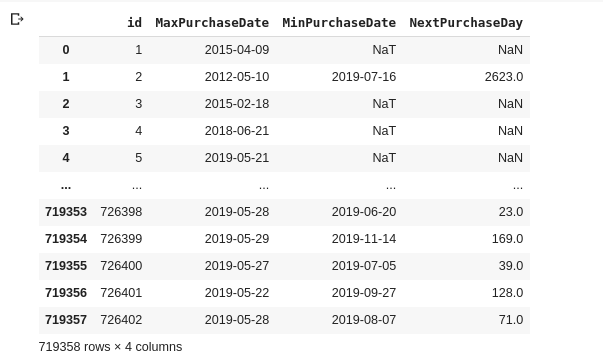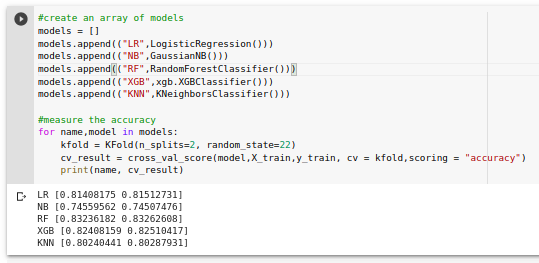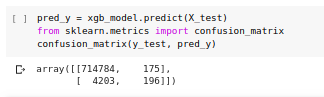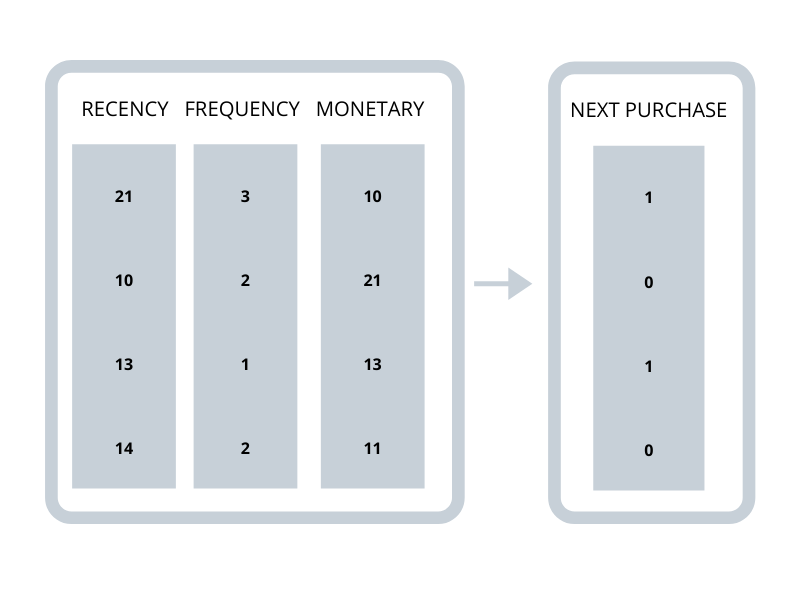# Predicting Next Purchase Using XGBoost and Python

###### Posted By : Aakash Chaddha | 29-Jan-2020

Predicting Next Purchase

Using XGBoost For Python App Development

For every company, whether big or small, customer retention is a hard pill. It is a green signal that indicates a company is doing good or not. Filtering fruitful customers from the haystack is always better for a company’s resources.

Using machine learning to calculate potential or long-term customers is now possible. We can train the model on our data to let it understand the minuscule variation on the customer data that can help produce an accurate prediction.

I will first explain to you all the terminologies that we need to understand before diving into the nitty-gritty of the codes. Code snippets will be available side-by-side.

# Terminology:

RFM Model: Recency, frequency, monetary Model used to track and filter a company's fruitful clients.

Recency: The more recently a customer made a purchase with a company, the more likely he or she is to keep the company and the brand in mind for subsequent purchases. The probability of engaging in future transactions with recent buyers is arguably higher as compared to consumers who have not bought from the company in months or even longer periods.

Frequency: The frequency of transactions of the customer may be affected by factors such as the type of product, the purchase price point and the need for replenishment or replacement.

Monetary Value: Monetary value stems from the cost-effectiveness of the customer's business expenses in the course of their transactions.

XGBOOST Model: XGBoost is a Machine Learning algorithm based on a decision-tree ensemble that uses a gradient boosting system. Artificial neural networks tend to outperform any other algorithms or systems when predicting problems involving unstructured data (images, text, etc.).

K-MEANS Clustering: K-means clustering is a method of vector quantization that is common for cluster analysis in data mining, originally from signal processing. The goal of the clustering of k-means is to divide n observations into k clusters in which each observation belongs to the cluster with the nearest mean, serving as a cluster prototype.

# Overview

We will be using customers to purchase data to predict their future repurchase chance within a given period of time. We will be needing their purchase date, item price, and customer id.

The following are the steps we may follow on this journey.

1. Data preprocessing
2. Training the model
3. Prediction on New Customers.

## Data Preprocessing:

Suppose a Gadget Company needs an ML model that can predict how much current customers are likely to repurchase again within the next 6 months. We currently have clients purchasing a history of 10 years from 2009-2019.We will be using only 9 years of data ( < 2019 ) to train the model. And use the last one year (2019) to do a perfect validation test on the model.

 ##Training data_tsc = df_data[(df_data.purchase_start_date < date(2018,1,1))].reset_index(drop=True) data_tsc_next = df_data[(df_data.purchase_start_date >= date(2018,1,1)) & (df_data.purchase_start_date < date(2019,1,1))].reset_index(drop=True)

We may use data_tsc and data_tsc_next to calculate the next purchase day.Replace all the NA data with 9999 for easy grouping.

As We are following the RFM model, let us calculate Recency, Frequency and Monetary value of each customer.

 tx_user = pd.DataFrame(data_tsc['id'].unique()) tx_user.columns = ['id'] tx_user

 ## For Recency tx_max_purchase = data_tsc.groupby('id').purchase_start_date.max().reset_index() tx_max_purchase.columns = ['id','MaxPurchaseDate']   tx_max_purchase['Recency'] = (tx_max_purchase['MaxPurchaseDate'].max() - tx_max_purchase['MaxPurchaseDate']).dt.days tx_user = pd.merge(tx_user, tx_max_purchase[['id','Recency']], on='id')

 ## For Frequency tx_frequency = data_tsc.groupby('id').purchase_start_date.count().reset_index() tx_frequency.columns = ['id','Frequency'] tx_user = pd.merge(tx_user, tx_frequency, on='id')

 ## For Monetary Value tx_revenue = data_tsc.groupby('id').price.sum().reset_index() tx_user = pd.merge(tx_user, tx_revenue, on='id')

Now we will make cluster of these three features and sort them with individually with the function order_cluster()

 def order_cluster(cluster_field_name, target_field_name,df,ascending):     df_new = df.groupby(cluster_field_name)[target_field_name].mean().reset_index()     df_new = df_new.sort_values(by=target_field_name,ascending=ascending).reset_index(drop=True)     df_new['index'] = df_new.index     df_final = pd.merge(df,df_new[[cluster_field_name,'index']], on=cluster_field_name)     df_final = df_final.drop([cluster_field_name],axis=1)     df_final = df_final.rename(columns={"index":cluster_field_name})     return df_final

Clustering Recency, Frequency, and Monetary Value:

 #clustering for Recency kmeans = KMeans(n_clusters=4) kmeans.fit(tx_user[['Recency']]) tx_user['RecencyCluster'] = kmeans.predict(tx_user[['Recency']]) tx_user = order_cluster('RecencyCluster', 'Recency',tx_user,False)

 #clustering for Frequency kmeans = KMeans(n_clusters=4) kmeans.fit(tx_user[['Frequency']]) tx_user['FrequencyCluster'] = kmeans.predict(tx_user[['Frequency']]) tx_user = order_cluster('FrequencyCluster', 'Frequency',tx_user,True)

 #clustering for Monetary Value kmeans = KMeans(n_clusters=4) kmeans.fit(tx_user[['Revenue']]) tx_user['RevenueCluster'] = kmeans.predict(tx_user[['Revenue']]) tx_user = order_cluster('RevenueCluster', 'Revenue',tx_user,True)

The last three consecutive transaction differences are added and the mean and standard deviation of their latest transaction difference is also calculated and added in the dataframe.We will include new columns “OverallScore” and “Segment”:

 #building overall segmentation tx_user['OverallScore'] = tx_user['RecencyCluster'] + tx_user['FrequencyCluster'] + tx_user['RevenueCluster'] #assign segment names tx_user['Segment'] = 'Low-Value' tx_user.loc[tx_user['OverallScore']>5,'Segment'] = 'Mid-Value' tx_user.loc[tx_user['OverallScore']>9,'Segment'] = 'High-Value'

The given dataframe is further grouped according to their next purchase day.

 tx_class['NextPurchaseDayRange'] = 3  ## less than 6 months tx_class.loc[tx_class.NextPurchaseDay>180,'NextPurchaseDayRange'] = 2 ## more than 6 months tx_class.loc[tx_class.NextPurchaseDay>365,'NextPurchaseDayRange'] = 1 ## more than 12 months

Till here, we have all the features and labels required for training our model.

## Training the Model:

For training our Model, splitting of data is required. Therefore,

 #train & test split tx_class = tx_class.drop('NextPurchaseDay',axis=1) X, y = tx_class.drop('NextPurchaseDayRange',axis=1), tx_class.NextPurchaseDayRange X_train, X_test, y_train, y_test = train_test_split(X, y, test_size=0.2, random_state=44)We will be using XGBoost Model to train our model.

 xgb_model = xgb.XGBClassifier().fit(X_train, y_train)

We can store our trained model:

 ### LOAD THE MODEL import pickle # save the model to disk filename = 'trainedmodel.sav' ##MODEL NAME pickle.dump(xgb_model, open(filename, 'wb')) ##SAVE MODEL # load the model from disk loaded_model = pickle.load(open(filename, 'rb')) xgb_model = loaded_model result = loaded_model.score(X_test, y_test) print(result) print('Accuracy of XGB classifier on training set: {:.2f}'       .format(loaded_model.score(X_train, y_train))) print('Accuracy of XGB classifier on test set: {:.2f}'       .format(loaded_model.score(X_test[X_train.columns], y_test)))

## Prediction on New Customers:

For testing our model on new customer data, I would suggest you to again repeat the step from the top, with these changes,

 data_tsc = df_data[(df_data.purchase_start_date >= date(2010,1,1)) & (df_data.purchase_start_date < date(2019,6,1))].reset_index(drop=True) data_tsc_next = df_data[(df_data.purchase_start_date >= date(2019,6,1)) & (df_data.purchase_start_date < date(2020,1,1))].reset_index(drop=True)

And follow each step as it is, Till the data splitting snippet.

Instead of training splitting, Do this.

 ## Testing ## testing data tx_class = tx_class.drop('NextPurchaseDay',axis=1) X_test, y_test = tx_class.drop('NextPurchaseDayRange',axis=1), tx_class.NextPurchaseDayRange

Load the pretrained model using the Load Model code in the Training the Model Section.

Use the following code to check your accuracy:

 print('Accuracy of XGB classifier on test set: {:.2f}'       .format(xgb_model.score(X_test, y_test)))

Confusion Matrix of the Prediction:Diagram of Feature and Labels for XGB Model.##### Related Tags